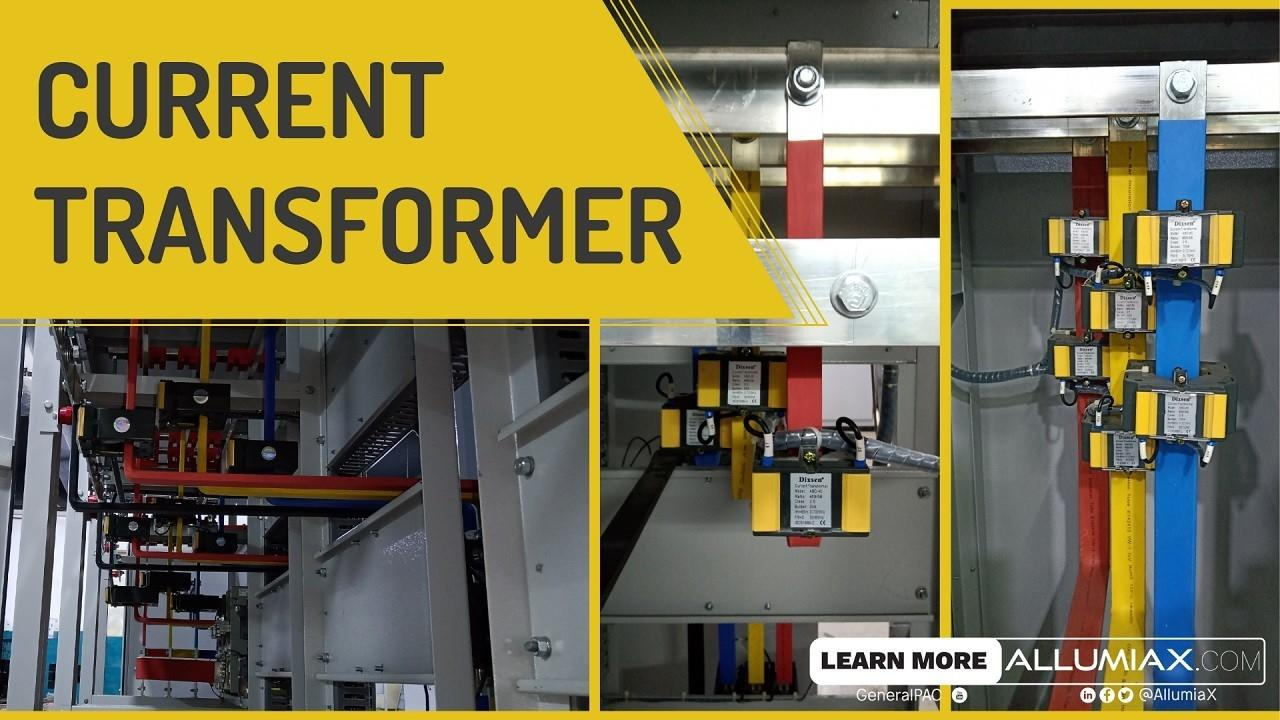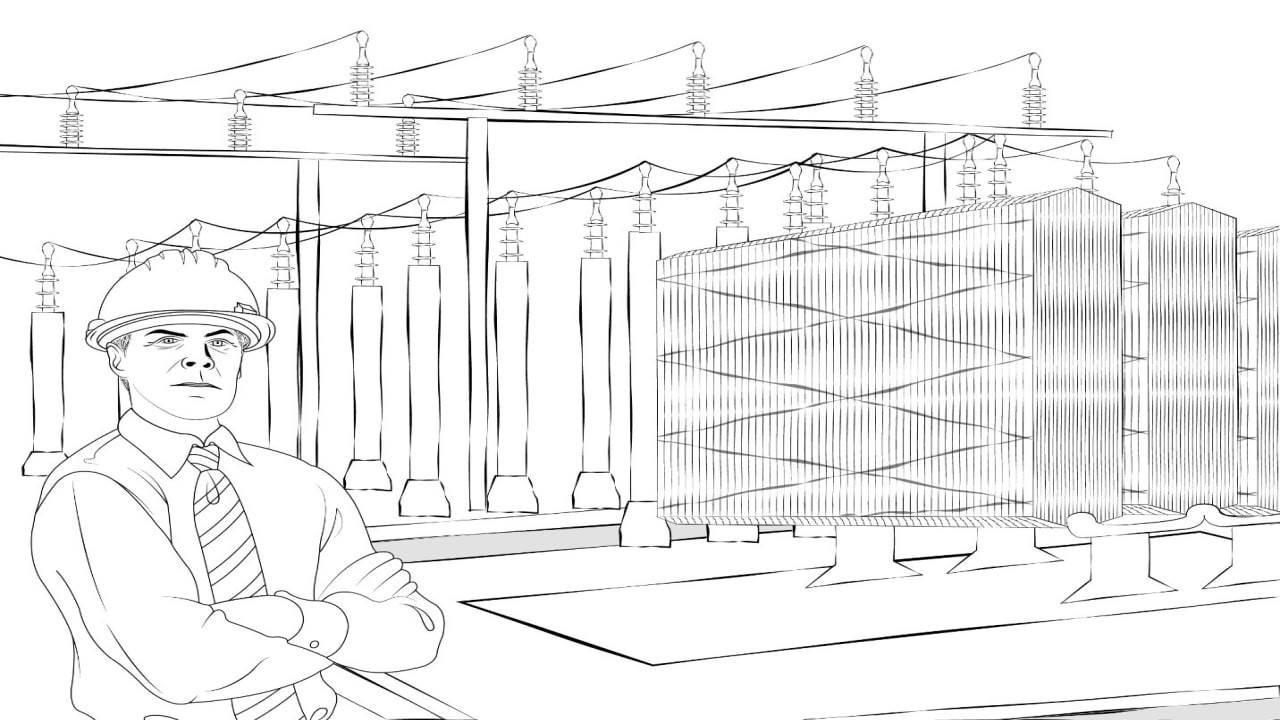(206) 552-8235 | [email protected]

# Current TransformerLast updated: Sep 25, 2020

This current transformer is an essential part of the power system. The basics of the current transformer including construction, applications, working principles are going to be discussed in this article. Moreover, some practical aspects such as grounding and connections of current transformer and associated errors will be examined comprehensively.We just launched our Power Systems Engineering Vlog series and in this series, we are going to talk about all sorts of various power system engineering studies and commentary. We will overview the different blogs written by AllumiaX. It's fun, it's lively, it's a video blog essentially and we hope you'll join us and benefit from it.

## What is Current Transformer & Why is it used?

Current Transformer is an 'instrumentation' transformer which steps down high values of currents to lower values.

As evident from their name, Instrumentation transformers are used to isolate the instrumentation devices from high voltages and currents to facilitate the measurement of electrical quantities.

Current Transformers are used extensively for measuring current and monitoring the operation of the power gridThe need for Current Transformer is justified by two reasons:

1. It isolates the protection system from high voltages and currents, resulting in the reduction of the size and cost of protection equipment.
2. The output of a Current Transformer is standard (i.e. 1A or 5A) which eliminates the need for protection equipment e.g. relays having diversified operating values.

## Construction of CT (Current Transformer):

The construction of a Current Transformer is very similar to a normal transformer. The core of the current transformer is built up with lamination of silicon steel.

A current transformer (CT) basically has a primary coil of one or more turns of heavy cross-sectional area. In some, the bar carrying high current may act as a primary. This is connected in series with the line carrying high current.

The secondary coil of the current transformer is made up of a large number of turns of fine wire having a small cross-sectional area. This is usually rated for 1A or 5A.

Hello there! On a related topic, we previously wrote a blog about Transformers — Its Working, Construction, Types, and Uses. If this peaks your interest, check it out and let us know what you think

## Working Principle:

Not only does the construction of current transformer similar to a regular transformer but the working principle is same as well.

The alternating current in the primary windings induces magnetic flux in the core which is transferred to the secondary windings and inducesalternating current there as well.

These transformers are basically step-up transformers i.e. stepping up a voltage from primary to secondary. Thus, the current reduces from primary to secondary.

## Classifications:

### Based on Function:

Measurement CT:

Current Transformer used for metering andindicating circuits are popularly termed as Measuring CTThey have a low saturation point. In case of a fault, the core will become saturated and the secondary current will not damage the measurement devices connected to it.

Protection CT:

Current Transformer used in conjunction with protective devices is termed as Protection CTThe purpose is to detect fault currents in the system and pass on the signal to relays. Since it operates on current values higher than its rated value, its core has a high saturation point.

### Based on Construction:

Bar Type Current Transformer:

This type of current transformer uses the actual cable or bus-bar of the main circuit as the primary winding, which is equivalent to a single turn. They are fully insulated from the high operating voltage.

Wound Current Transformer:

The transformers primary winding is physically connected in series with the conductor that carries the measured current flowing in the circuit.

Toroidal/Window Current Transformer:

These do not contain a primary winding. Instead, the line that carries the current flowing in the network is threaded through a window or hole in the toroidal transformer. Some current transformers have a "split core" which allows it to be opened, installed, and closed, without disconnecting the circuit to which they are attached.

## Connections of CTs:

A CT is quite simple to connect in a single phase system, but for three phase system, there are 3 CTs which can be connected in two ways:

### Star (Wye) Connected:

In case of star connection, the polar side of the Current Transformers is connected to the equipment i.e. relay and the non-polar sides are shorted and then grounded. The neutral side may or may not be present in the three phase system.

### Delta Connected:

For a delta connection, the CTs are connected to each other in delta fashion, but the polarity of the CTs is kept in mind while making the connections.

It is a normal practice to connect the CTs in delta if it is on the transformer side connected in wye and vice-versa.

### Polarity of CT:

Like any other transformer, a CT also has a polarity. Polarity refers to the instantaneous direction of the primary current with respect to the secondary current and is determined by the way transformer leads are brought out of the case.

All current transformers are subtractive polarity. CT polarity is sometimes indicated with an arrow, these CTs should be installed with the arrow pointing in the direction of current flow.

It is very important to observe correct polarity when installing and connecting current transformers to power metering and protective relays.

### Grounding of CT:

The grounding of the current transformer is very important for the safety and correct operation of the protective relays.

As per the grounding standard of the current transformer, the current transformer secondary circuit should be connected to the station ground at only onepoint. This holds true irrespective of the number of current transformer secondary winding connected to the circuit.

### Burden of a CT:

Current transformer burden is defined as the load connected across its secondary. It is generally expressed in VA(volt-ampere).

In short, the connecting wires and the connected meter form the load of the current transformer. In technical terms this is called load in VA. This load influences the accuracy of the current transformer. In the design of the current transformer, internal losses and the external load of the current transformer are taken into account.

The burden is expressed in VA by multiplying the secondary current with the voltage drop across the burden (load) of the CTA current transformer is divided into classes on the basis of accuracy which, in turn, depends on the burden of the CT.

### CT Ratio:

The CT ratio is the ratio of primary current input to secondary current output at full load. For example, a CT with a ratio of 100:5 is rated for 100 primary amps at full load and will produce 5 amps of secondary current when 100 amps flow through the primary.

Transformer Ratio =
Primary CurrentSecondary Current

### Errors in a CT:

The current transformer has two errors – ratio error and a phase angle error.

#### Current Ratio Errors

It is mainly due to the energy component of excitation current and is given as

Ratio Error =
KtIs - IpIp

Where Ip is the primary current, Kt is the turn ratio and Is is the secondary current.

#### Phase Angle Error

In an ideal current transformer, the vector angle between the primary and reversed secondary current is zero. But in an actual current transformer, there is a phase difference between the primary and the secondary current because the primary current has also supplied the component of exciting current. Thus, the difference between the two phases is termed as a phase angle error.

### Phasor diagrams of an ideal and actual CT: ​

The ideal current transformer may be defined in which any primary condition is reproduced in the secondary circuit in the exact ratio and phase relationship. The phasor diagram for an ideal current transformer is shown in Figure 1.

In an actual transformer, the windings have resistance and reactance and the transformer also has magnetizing and loss component of current to maintain the flux (see Figure 2). Therefore, in an actual transformer the ratio of current is not equal to the turns ratio and there is also a phase difference between the primary current and the secondary currents reflected back on the primary side. Consequently, we have ratio error and phase angle error.

Where:

Kn = turns ratio = number of secondary winding turns/number of primary winding turns,

Rs, Xs = resistance and reactance respectively of the secondary winding,

Rp, Xp = resistance and reactance respectively of the primary winding,

Ep, Es = primary and secondary induced voltages respectively,

Tp, Ts = number of primary winding and secondary winding turns respectively,

Ip, Is = primary and secondary winding currents respectively,

θ = phase angle of the transformer

Φm = working flux of the transformer

δ = angle between secondary induced voltage and secondary current,

I= exciting current,

Im = magnetizing component of exciting current

Il = loss component of exciting current,

α = angle between Io and Φm

You will emerge with the knowledge in principles, working, applications, sizing of CT that empowers you to have a strong grip on the fundamentals of the Current Transformer. Check out Current Transformer Fundamentals Course in which we briefly discussed "Current Transformer Equivalent Circuit Mode".

## Model of a CT:

The Current Transformer’s modelling is just the same as any other transformer. The model of CT is as below:

X1 = Primary leakage reactance

R1 = Primary winding resistance

X2 = Secondary leakage reactance

Z0 = Magnetizing impedance

R2 = Secondary winding resistance